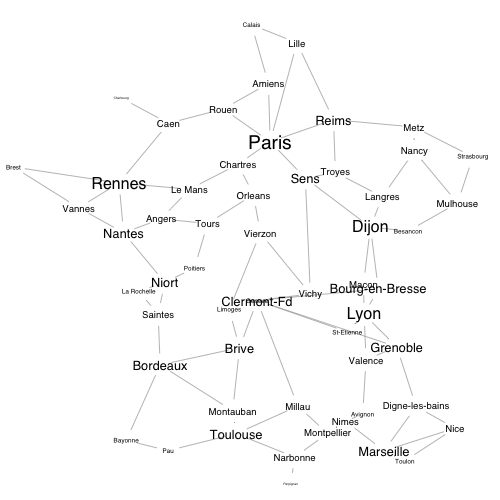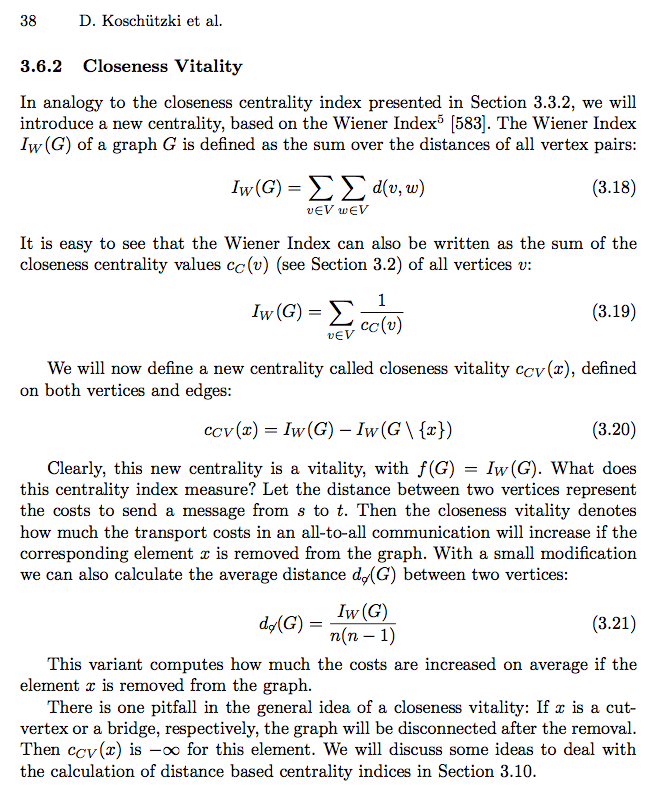## From networkx to igraph

This note translates the code from an interesting blog post (in French) from Python to R. The code includes a function to compute closeness vitality with the igraph package.

## Data generation

The blog post, which was written by Serge Lhomme, is based on a network made of French cities connected by highways as its data example. The network is unweighted and undirected, and has a density of around 0.05.

The Python code to generate the data example cannot be directly re-used in R, because R cannot read Python arrays. The igraph library, however, can evaluate a literal sequence of graph edges, and generate a graph from these:

The second line of the data generation code creates exactly the same sequence of edges as the Python codes does, whereas the last line assigns vertex names to the graph. The "+1" part of that last line is due to the fact that R indexation starts at 1 instead of starting at 0 as it does in Python.

The rest of this note is organised around the centrality measures that can be computed to describe the graph. Computing centrality measures is fairly similar in networkx and in igraph, although one has to be careful to set the weighting and normalizing options identically to get matching results.

## Degree and betweenness

The first centrality measures mentioned in the blog post are degree and betweenness, which are stored in objects deg and bet. The code to generate them in R assigns them directly to the graph nodes, instead of storing them as separate objects:

The Python code does not include a plot of the data, which is a bit of a shame. Let's fix that by geocoding the nodes and by plotting them at sizes that reflect their degree centrality:## Vulnerability

The blog post continues by suggesting a simple way to "stress test" a graph: for each node in the graph, remove that node, compute the average shortest path length of the resulting graph, and compare it to that same measure in the initial graph.

This measure is also easy to implement with igraph, thanks to the mean_distance function:

The loop above can be turned into an igraph-style function that returns a vector of the measure along with the names of the vertices to which each measurement corresponds:

The only "sensitive" part of the code is where we control for the possibility that removing a node creates an unconnected graph (which will happen if the node is a cut-vertex—more on that later). In that case, the vulnerability measure is set to NA, since the distance matrix on which it is based includes some infinite values.

## Closeness vitality

The last centrality measure mentioned in the blog post is closeness vitality, which is not implemented in igraph. Let's take a look at the source of the function, which is mentioned in the documentation of its networkx implementation:The text explains that, to compute the closeness vitality of node $x$ in graph $g$, you need to compute two Wiener indexes: one for the initial graph $g$, and one for that same graph after node $x$ has been removed (Equation 3.20). The Wiener index is simply the sum of the distance matrix of a graph (Equation 3.18), which is trivial to implement through igraph.

The text also explains that closeness vitality will be equal to $-\infty$ when the node for which it is being computed is crucial to keeping the graph connected. The networkx implementation of closeness vitality avoids that issue by removing infinite values from the distance matrix, which will also be our strategy so that we can match the networkx results as exactly as possible.

Adding a closeness vitality function to igraph takes only a few lines of code, and getting the $R^2$ value mentioned in the blog post is also trivial:

The code from this note is available as a Gist, which also contains the Python code that I translated. For additional connectivity tests, see Serge Lhomme's NetSwan package, which is discussed in this other blog post (also in French).

The code to implement the closeness vitality function has been submitted as a possible addition to the igraph package.

• First published on October 31st, 2015